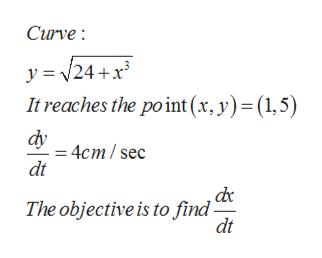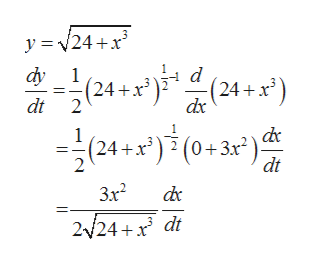# A particle moves along the curve below. y=(24+(x^3))^(1/2)As it reaches the point (1,5), the y-coordinate is increasing at a rate of 4cm/s. How fast is the x-coordinate of the point changing at that instant?

Question
33 views

A particle moves along the curve below.

y=(24+(x^3))^(1/2)

As it reaches the point (1,5), the y-coordinate is increasing at a rate of 4cm/s. How fast is the x-coordinate of the point changing at that instant?

check_circle

Step 1

A particle moves along the curve f(x). As it reaches the point (1,5), the y-coordinate is increasing at a rate of 4 cm/s. How fast is the x-coordinate of the point changing at that instant.

Step 2

Given informationhelp_outlineImage TranscriptioncloseCurve: y= 24+x It reaches the point (x, y) = (1,5) dy - 4cm/sec dt ,dc The objective is to find- dt fullscreen
Step 3

Differentiate the curve w....help_outlineImage Transcriptionclosey 24+x d dy 1 (24 + x) (24+x) dt 2 dx 1 (24+x) (0+3x2)cd¢ dt 3x2 dc 2/24 x dt fullscreen

### Want to see the full answer?

See Solution

#### Want to see this answer and more?

Solutions are written by subject experts who are available 24/7. Questions are typically answered within 1 hour.*

See Solution
*Response times may vary by subject and question.
Tagged in

### Functions